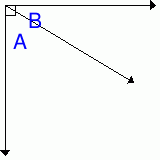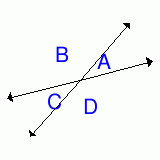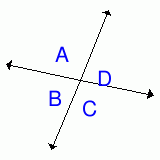MathScore EduFighter is one of the best math games on the Internet today. You can start playing for free!

## Angle Measurements - Sample Math Practice Problems

The math problems below can be generated by MathScore.com, a math practice program for schools and individual families. References to complexity and mode refer to the overall difficulty of the problems as they appear in the main program. In the main program, all problems are automatically graded and the difficulty adapts dynamically based on performance. Answers to these sample questions appear at the bottom of the page. This page does not grade your responses.

See some of our other supported math practice problems.

### Complexity=1, Mode=comp

Solve for the missing angle(s).
Figures are not drawn to scale.

1.   What is the complement of an angle that measures 58°?    °
2.   If m∠A = 41°, what is m∠B?m∠B = °

### Complexity=1, Mode=supp

Solve for the missing angle(s).
Figures are not drawn to scale.

 1.   What is the supplement of an angle that measures 22°?    ° 2.   ∠A and ∠B are supplementary angles. If m∠B= 97°,what is m∠A?    °

### Complexity=1, Mode=vert

Solve for the missing angle(s).
Figures are not drawn to scale.

1.   A and ∠B are vertical angles. If m∠A= 5°,
what is m∠B?    °
2.   In the figure below, two lines intersect to form ∠A,
B, ∠C, and ∠D.If m∠A = 31°, what is m∠C?      °

### Complexity=1

Solve for the missing angle(s).
Figures are not drawn to scale.

1.   What is the supplement of an angle that measures 73°?    °
2.   In the figure below, two lines intersect to form ∠A,
B, ∠C, and ∠D.If m∠D = 82°, find the missing angles.
m∠A = °
m∠B = °
m∠C = °

### Complexity=1, Mode=comp

Solve for the missing angle(s).
Figures are not drawn to scale.

1What is the complement of an angle that measures 58°?    °
Solution
Complementary angles add up to 90°.
2If m∠A = 41°, what is m∠B?m∠B = °
Solution
A and ∠B are complementary angles which means they add up to 90°.
 m∠A + m∠B = 90° m∠B = 90° - m∠A m∠B = 90° - 41° = 49°

### Complexity=1, Mode=supp

Solve for the missing angle(s).
Figures are not drawn to scale.

1What is the supplement of an angle that measures 22°?    °
Solution
Supplementary angles add up to 180°.
2A and ∠B are supplementary angles. If m∠B= 97°,
what is m∠A?
°
Solution
B and ∠A are supplementary angles which means they add up to 180°.
 m∠B + m∠A = 180° m∠A = 180° - m∠B m∠A = 180° - 97° = 83°

### Complexity=1, Mode=vert

Solve for the missing angle(s).
Figures are not drawn to scale.

1A and ∠B are vertical angles. If m∠A= 5°,
what is m∠B?
°
Solution
A and ∠B are vertical angles which means they are equal.
m∠A = m∠B = 5°
2In the figure below, two lines intersect to form ∠A,
B, ∠C, and ∠D.If m∠A = 31°, what is m∠C?
°
Solution
A and ∠C are vertical angles which means they are equal.
m∠A = m∠C = 31°

### Complexity=1

Solve for the missing angle(s).
Figures are not drawn to scale.

1What is the supplement of an angle that measures 73°?    °
Solution
Supplementary angles add up to 180°.
2In the figure below, two lines intersect to form ∠A,
B, ∠C, and ∠D.If m∠D = 82°, find the missing angles.

m∠A = °
m∠B = °
m∠C = °
Solution

D and ∠A are supplementary angles which means they add up to 180°.
 m∠D + m∠A = 180° m∠A = 180° - m∠D m∠A = 180° - 82° = 98°

D and ∠B are vertical angles which means they are equal.
m∠D = m∠B = 82°

D and ∠C are supplementary angles which means they add up to 180°.
 m∠D + m∠C = 180° m∠C = 180° - m∠D m∠C = 180° - 82° = 98°